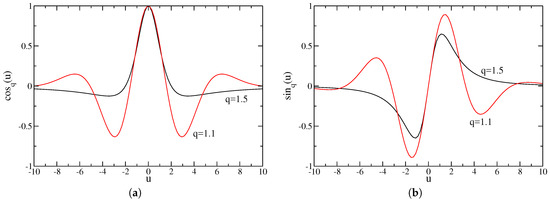Next Article in Journal
Next Article in Special Issue
Nonlinear Wave Equations Related to Nonextensive Thermostatistics
Previous Article in Journal / Special Issue
Intermittent Motion, Nonlinear Diffusion Equation and Tsallis Formalism
Open AccessReview

# Nonlinear q-Generalizations of Quantum Equations: Homogeneous and Nonhomogeneous Cases—An Overview

1
Centro Brasileiro de Pesquisas Físicas and National Institute of Science and Technology for Complex Systems, Rua Xavier Sigaud 150, Urca, Rio de Janeiro 22290-180, Brazil
2
Santa Fe Institute, 1399 Hyde Park Road, Santa Fe, NM 87501, USA
*
Author to whom correspondence should be addressed.
Entropy 2017, 19(1), 39; https://doi.org/10.3390/e19010039
Received: 15 December 2016 / Revised: 12 January 2017 / Accepted: 16 January 2017 / Published: 21 January 2017
Recent developments on the generalizations of two important equations of quantum physics, namely the Schroedinger and Klein–Gordon equations, are reviewed. These generalizations present nonlinear terms, characterized by exponents depending on an index q, in such a way that the standard linear equations are recovered in the limit $q → 1$ . Interestingly, these equations present a common, soliton-like, traveling solution, which is written in terms of the q-exponential function that naturally emerges within nonextensive statistical mechanics. In both cases, the corresponding well-known Einstein energy-momentum relations, as well as the Planck and the de Broglie ones, are preserved for arbitrary values of q. In order to deal appropriately with the continuity equation, a classical field theory has been developed, where besides the usual $Ψ ( x → , t )$ , a new field $Φ ( x → , t )$ must be introduced; this latter field becomes $Ψ * ( x → , t )$ only when $q → 1$ . A class of linear nonhomogeneous Schroedinger equations, characterized by position-dependent masses, for which the extra field $Φ ( x → , t )$ becomes necessary, is also investigated. In this case, an appropriate transformation connecting $Ψ ( x → , t )$ and $Φ ( x → , t )$ is proposed, opening the possibility for finding a connection between these fields in the nonlinear cases. The solutions presented herein are potential candidates for applications to nonlinear excitations in plasma physics, nonlinear optics, in structures, such as those of graphene, as well as in shallow and deep water waves. View Full-Text
Show FiguresFigure 1

MDPI and ACS Style

Nobre, F.D.; Rego-Monteiro, M.A.; Tsallis, C. Nonlinear q-Generalizations of Quantum Equations: Homogeneous and Nonhomogeneous Cases—An Overview. Entropy 2017, 19, 39.